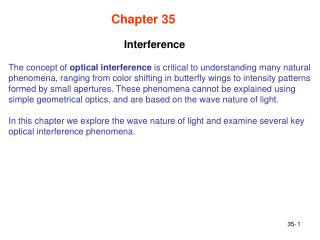DownloadDownload PresentationInterference

# Interference

Download Presentation## Interference

- - - - - - - - - - - - - - - - - - - - - - - - - - - E N D - - - - - - - - - - - - - - - - - - - - - - - - - - -
##### Presentation Transcript

1. Interference Chapter 35 The concept of optical interference is critical to understanding many natural phenomena, ranging from color shifting in butterfly wings to intensity patterns formed by small apertures. These phenomena cannot be explained using simple geometrical optics, and are based on the wave nature of light. In this chapter we explore the wave nature of light and examine several key optical interference phenomena. 35-

2. Light as a Wave Fig. 35-2 Huygen’s Principle: All points on a wavefront serve as point sources of spherical secondary wavelets. After time t, the new position of the wavefront will be that of a surface tangent to these secondary wavelets. 35-

3. Law of Refraction Index of Refraction: Law of Refraction: Fig. 35-3 35-

4. Wavelength and Index of Refraction Fig. 35-4 The frequency of light in a medium is the same as it is in vacuum Since wavelengths in n1 and n2 are different, the two beams may no longer be in phase 35-

5. Rainbows and Optical Interference Fig. 35-5 The geometrical explanation of rainbows given in Ch. 34 is incomplete. Interference, constructive for some colors at certain angles, destructive for other colors at the same angles is an important component of rainbows 35-

6. Diffraction Fig. 35-7 For plane waves entering a single slit, the waves emerging from the slit start spreading out, diffracting. 35-

7. Young’s Experiment Fig. 35-8 For waves entering a two slit, the emerging wavesinterfere and form an interference (diffraction) pattern. 35-

8. Locating Fringes Path Length Difference: Fig. 35-10 The phase difference between two waves can change if the waves travel paths of different lengths. What appears at each point on the screen is determined by the path length difference DL of the rays reaching that point. 35-

9. Locating Fringes Maxima-bright fringes: Minima-dark fringes: Fig. 35-10 35-

10. Coherence Two sources to produce an interference that is stable over time, if their light has a phaserelationship that does not change with time: E(t)=E0cos(wt+f) Coherent sources: Phasefmust be well defined and constant. When waves from coherent sources meet, stable interference can occur. Sunlight is coherent over a short length and time range. Since laser light is produced by cooperative behavior of atoms, it is coherent of long length and time ranges Incoherent sources: fjitters randomly in time, no stable interference occurs 35-

11. Intensity in Double-Slit Interference E2 E1 Fig. 35-12 35-

12. Proof of Eqs. 35-22 and 35-23 Fig. 35-13 Eq. 35-22 Eq. 35-23 35-

13. hitt In a Young's double-slit experiment the center of a bright fringe occurs wherever waves from the slits differ in the distance they travel by a multiple of: A. a fourth of a wavelength B. a half a wavelength C. a wavelength D. three-fourths of a wavelength E. none of the above

14. Combining More Than Two Waves E4 E3 E E2 E1 • In general, we may want to combine more than two waves. For example, there may be more than two slits. • Procedure: • Construct a series of phasors representing the waves to be combined. Draw them end to end, maintaining proper phase relationships between adjacent phasors. • Construct the sum of this array. The length of this vector sum gives the amplitude of the resulting phasor. The angle between the vector sum and the first phasor is the phase of the resultant with respect to the first. The projection of this vector sum phasor on the vertical axis gives the time variation of the resultant wave. 35-

15. Interference from Thin Films Fig. 35-15 35-

16. Reflection Phase Shifts n1 > n2 n1 < n2 n1 n1 n2 n2 Fig. 35-16 Reflection Reflection Phase Shift Off lower index 0 Off higher index 0.5 wavelength 35-

17. Equations for Thin-Film Interference Fig. 35-17 • Three effects can contribute to the phase difference between r1 and r2. • Differences in reflection conditions • Difference in path length traveled. • Differences in the media in which the waves travel. One must use the wavelength in each medium (l / n), to calculate the phase. ½ wavelength phase difference to difference in reflection of r1 and r2 35-

18. Film Thickness Much Less Than l r2 r1 If L much less than l, for example L < 0.1l, than phase difference due to the path difference 2L can be neglected. Phase difference between r1 and r2will always be ½ wavelength  destructive interference  film will appear dark when viewed from illuminated side. 35-

19. Color Shifting by Morpho Butterflies and Paper Currencies Fig. 35-19 For the same path difference, different wavelengths (colors) of light will interfere differently. For example, 2L could be an integer number of wavelengths for red light but a half integer wavelengths for blue. Furthermore, the path difference 2L will change when light strikes the surface at different angles, again changing the interference condition for the different wavelengths of light. 35-

20. Problem Solving Tactic 1: Thin-Film Equations Equations 35-36 and 35-37 are for the special case of a higher index film flanked by air on both sides. For multilayer systems, this is not always the case and these equations are not appropriate. What happens to these equations for the following system? L r2 r1 n1=1 n2=1.5 n3=1.7 35-

21. Michelson Interferometer Fig. 35-20 For each change in path by 1l, the interference pattern shifts by one fringe at T. By counting the fringe change, one determines Nm- Na and can then solve for L in terms of l and n. 35-